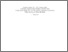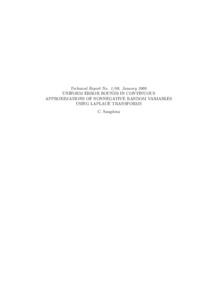Title:

# Uniforms Error Bounds in Continuous Approximations of Nonnegative Random Variables Using Laplace Transforms

Sangüesa, Carmen (2008) Uniforms Error Bounds in Continuous Approximations of Nonnegative Random Variables Using Laplace Transforms. Technical Report. Concordia University. Department of Mathematics & Statistics, Montreal, Quebec.Preview
Text (application/pdf)
1_08_Sanguesa.pdf - Published Version
275kB

## Abstract

In this work we deal with approximations for distribution functions of nonnegative random variables. More specifically, we construct continuous approximants using an acceleration technique over a well-know inversion formula for Laplace transforms. We give uniform error bounds using a
representation of these approximations in terms of gamma-type operators. We apply our results to certain mixtures of Erlang distributions which contain the class of continuous phase-type distributions.

Divisions: Concordia University > Faculty of Arts and Science > Mathematics and Statistics Monograph (Technical Report) Sangüesa, Carmen Department of Mathematics & Statistics. Technical Report No. 1/08 Concordia University. Department of Mathematics & Statistics Concordia University January 2008 Uniform distance; Laplace transform; gamma distribution; phase-type distribution 6684 DIANE MICHAUD 03 Jun 2010 20:13 18 Jan 2018 17:29

## References:

Adell, J. A. and de la Cal, J. (1993). On the uniform convergence of normalized Poisson mixtures to their mixing distribution, Statist. Probab. Lett. 18, 227-232.

Adell, J. A. and de la Cal, J. (1994). Approximating gamma distributions by normalized negative binomial distributions, J. Appl. Probab. 31, 391-400.

Adell J. A. and Sangüesa C. (1999). Direct and converse inequalities for positive linear operators on the positive semi-axis, J. Austral. Math. Soc. Ser. A 66, 90-103.

Alzer, H. (1997) On some inequalities for the gamma and psi functions, Math. Comp., 66, 373-389.

Asmussen, S. (2000). Ruin probabilities, World Scientific, Singapore.

Embrechts, P., Grübel, R. and Pitts, S. M. (1993) Some applications of the fast Fourier transform algorithm in insurance mathematics, Statist. Neerlandica, 47, 59-75.

Feller, W. (1971). An Introduction to Probability Theory and its Applications, Vol II, 2nd. edition. Wiley, New York.

Grübel, R. and Hermesmeier, R. (2000). Computation of compound distributions II: discretization errors and Richardson extrapolation. Astin Bull. 30, 309-331.

Hipp, C. (2006). Speedy convolution algorithms and Panjer recursions for phase-type distributions. Insurance Math. Econom. 38, 176

Latouche, G. and Ramaswami, V. (1999). Introduction to Matrix Analytic Methods in Stochastic Modelling, ASA-SIAM, Philadelphia.

Maier, R.S. (1991) The algebraic construction of phase-type distributions, Comm. Statist. Stochastic Models, 7, 573-602.

O'Cinneide, C. A. (1999) Phase-type distributions: open problems and a few properties., Comm. Statist. Stochastic Models, 15, 731-757.

Qi, F., Cui, R., Chen, C., Guo, B. (2005) Some completely monotonic functions involving polygamma functions and an application. J. Math. Anal. Appl. 310 , 303-308.

Sangüesa, C. (2007). Error bounds in approximations of compound distributions using gamma-type operators, To appear in Insurance Math. Econom.

Sundt, B. (2002). Recursive evaluation of aggregate claims distributions, Insurance Math. Econom. 30, 297-322.

Willmot, G. E. and Woo, J. K. (2007). On the class of Erlang mixtures with risk theoretical applications, N. Am. Actuar. J. 11, 99-105
All items in Spectrum are protected by copyright, with all rights reserved. The use of items is governed by Spectrum's terms of access.

Repository Staff Only: item control pageResearch related to the current document (at the CORE website)
Back to top